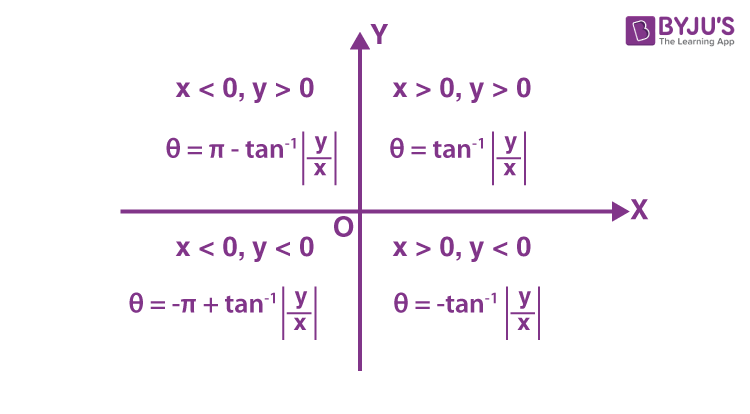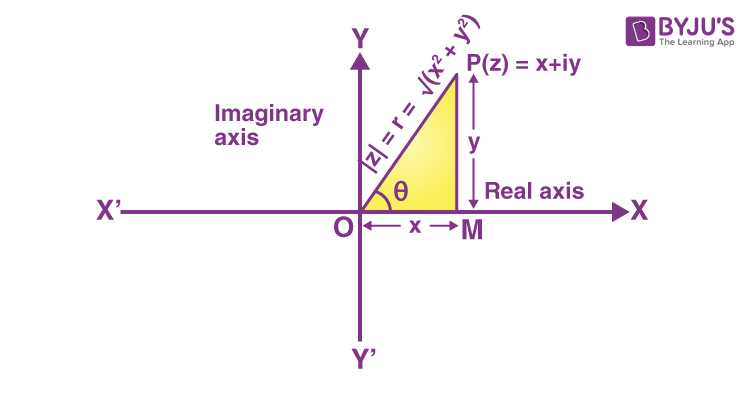# Complex Numbers JEE Notes

Complex numbers JEE notes contain all the formulas and important points which are very important for the JEE entrance exam. Students can expect 2-3 questions from this topic for any entrance exam. It is not possible to revise complete books when the exams are round the corner. So, we have come up with complex numbers notes for IIT JEE. You can also download complex numbers IIT JEE notes pdf for free. Complex numbers IIT JEE notes will definitely help students to have a quick complete revision.

A complex number is a number expressed in the form z = a+ib, where a and b are real numbers and i = √-1 (iota). The real part of z is given by Re(z) = a and the imaginary part of z is given by Im(z) = b. The complex number is purely imaginary, if Re(z) = 0. The complex number is purely real, if Im(z) = 0. A complex number z can also be defined as an ordered pair of real numbers and can be denoted by (a, b). If we denote z = (a, b), then the real part of z is a and the imaginary part of z is b.

## Complex Numbers IIT JEE Notes

### Algebraic Operations with Complex Numbers

1. Addition: (a+ib) + (c+id) = (a+c) + i(b+d)

2. Subtraction: (a+ib) – (c+id) = (a-c) + i(b-d)

3. Multiplication: (a+ib)(c+id) = (ac-bd) + i(ad+bc)

4. Division: (a+ib)/(c+id) = (ac + bd)/(c2 + d2) + i (bc – ad)/(c2 + d2) (at least one of c and d is non zero)

Properties of Algebraic Operations

a) z1z2 = z2z1

b) z1 + z2 = z2 + z1

c) (z1 + z2) + z3 = z1 + (z2 + z3)

d) (z1z2)z3 = z1(z2z3)

e) z+0 = z and z.1 = 1.z = z. (0 and 1 are the identity elements for addition and multiplication respectively.)

f) Additive inverse of z is -z.

g) Multiplicative inverse of z is 1/z.

h) z1(z2+z3) = z1z2 + z1z3 and (z2+z3) z1 = z2z1 + z3z1

### Conjugate Of A Complex Number

The conjugate of complex number z = a+ib is given by $\overline{z}=a-ib$.

Important Properties of Conjugate of a Complex Number

(1) $\bar{\bar{z}}= z$

(2) ${{z}_{1}}={{z}_{2}}\Leftrightarrow {{\overline{z}}_{1}}={{\overline{z}}_{2}}$

(3) $\overline{\left( \frac{{{z}_{1}}}{{{z}_{2}}} \right)}=\frac{{{\overline{z}}_{1}}}{{\overline{z}_{2}}}$, if z2 ≠ 0

(4) $z+\overline{z}=2\,{Re}(z)$

(5) $z-\overline{z}=2i\,{Im}\,(z)$

(6) $z=\overline{z}\Leftrightarrow z$ is purely real

(7) $z+\overline{z}=0\Leftrightarrow z$ is purely imaginary.

(8) $z\overline{z}={{[Re\,(z)]}^{2}}+{{[Im(z)]}^{2}}=a^{2}+b^{2}=\left | z \right |^{2}$

(9) $\overline{{{z}_{1}}+{{z}_{2}}}={{\overline{z}}_{1}}+{{\overline{z}}_{2}}$

(10) $\overline{{{z}_{1}}-{{z}_{2}}}={{\overline{z}}_{1}}-{{\overline{z}}_{2}}$

(11) $\overline{{{z}_{1}}{{z}_{2}}}={{\overline{z}}_{_{1}}}{{\overline{z}}_{_{2}}}$

(12) $\overline{re^{i\theta }}=re^{-i\theta }$

### Modulus of a complex number

If z = x+iy, then modulus of z is denoted by |z| = √(x2+y2).

|z| is a non negative real number.

Important Properties of Modulus of a Complex Number

1. |z| ≥ 0 and |z| = 0 iff x = 0 and y = 0 (z = 0)

2. -|z| ≤ Re(z) ≤ |z|

3. |z| = $\left | \overline{z} \right |$ = |-z| = $\left | -\overline{z} \right |$

4. $z\overline{z} =\left | z \right |^{2}$

5. z-1 = $\frac{\overline{z}}{\left | z \right |^{2}}$

6. |z2| = |z|2 or |zn| = |z|n, n belongs to N.

7. |z1z2….zn| = |z1||z2|….|zn|

8. |z1 + z2| ≤ |z1| + |z2|

9. |z1 – z2| ≥ ||z1| – |z2||

10. |z1 + z2|2 + |z1 – z2|2 = 2(|z1|2 + |z2|2)

11. |z1 + z2|2 = |z1|2 + |z2|2 + 2Re($z_{1}\overline{z_{2}}$)

### Integral Powers of iota (i)

We know that i = √-1.

• i2 = -1
• i3 = -i
• i4 = 1
• The sum of four consecutive powers of i is zero.

### Square Root of a Complex Number

Let z = x + iy then

$\sqrt{x+iy}=\left\{ \begin{matrix} \pm \left[ \sqrt{\frac{|z|+x}{2}}+i\sqrt{\frac{|z|-x}{2}} \right]if\,y\,>\,0 \\ \pm \left[ \sqrt{\frac{|z|+x}{2}}-i\sqrt{\frac{|z|-x}{2}} \right]if\,y\,<\,0 \\ \end{matrix} \right.$

Where |x| = $\sqrt{{{x}^{2}}+{{y}^{2}}}\,$

(i) $\sqrt{x+iy}+\sqrt{x-iy}=\sqrt{2|z|+2x}$

(ii) $\sqrt{x+iy}-\sqrt{x-iy}=i\sqrt{2|z|-2x}$

(iii) $\sqrt{i}=\pm \left( \frac{1+i}{\sqrt{2}} \right)\,and\,\sqrt{-i}=\pm \left( \frac{1-i}{\sqrt{2}} \right)$

### Amplitude of a Complex Number

If z = x+iy, then amplitude or argument of z is given by amp(z) = tan-1(y/x).

To find the argument of z, find the quadrant in which the complex number belongs and then find the angle θ and amplitude using the figure below.Note: (a) the principal value of any complex number lies between -π <θ <π.

b) Amplitude of any complex number is a many valued function. If θ is the argument of a complex number then (2nπ + θ) is also the argument of complex number.

c) If the complex number is multiplied by i (iota), then the amplitude will be increased by π/2 and if it is multiplied by -i, then the amplitude will be decreased by π/2.

d) Argument of 0 is not defined.

e) In first and second quadrants, amplitude is always positive. In the third and fourth quadrant, amplitude is always negative.

### Representation of a Complex Number

Cartesian Representation:

The complex number z = x+iy is represented by a point P whose coordinates are referred to the rectangular axis xox’ and yoy’, which are called real and imaginary axes, respectively. A complex number z is represented by a point in a plane. There exists a complex number corresponding to every point in this plane. Such a plane is called the Argand plane or Argand diagram.(i) Distance of any complex number from the origin is called the modulus of complex number. Thus

$\left | z \right |=\sqrt{x^{2}+y^{2}}$.

(ii) Angle of any complex number with positive direction of the x-axis is called amplitude or argument of z. amp(z) = arg(z) = θ = tan-1(y/x).

Polar Form: If z = x+iy is a complex number, then the polar form is given by

z = r(cos θ + i sin θ), where x = r cos θ, y = r sin θ and r = √(x2+y2) = |z|.

Exponential Form: If z = x+iy is a complex number, then z = reis the exponential form. Here r is the modulus and θ is the amplitude of the complex number.

Vector Representation: If z = x+iy is a complex number, then its vector representation is given by $z=\overrightarrow{OP}$.

#### De-Moivre’s Theorem

If n ∈ Z(set of integers), then (cos θ + i sin θ)n = (cos nθ + i sin nθ)

Or (e)n = einθ

De Moivre’s Theorem is used to find the roots of complex numbers.

#### Cube Roots of Unity

The three cube roots of unity are 1, (-½ + √3i/2) and (-½ – √3i/2). 1 is a real number and the other two are complex conjugate numbers and they are called imaginary cube roots of unity.

We write ω = -½ + √3i/2

Note: a) 1+ω+ω2 = 0

b) ω3 = 1

c) ω3n = 1

d) ω3n+1 = ω

e) ω3n+2 = ω2

#### Geometry of Complex Numbers

a) Distance formula:

Let z1 = x1+iy1 and z2 = x2+iy2 be two complex numbers represented by points P and Q respectively in Argand Plane, then PQ = √[(x2 – x1)2 + (y2-y1)2]

= |(x2-x1) + i(y2-y1)|

= |z2-z1|

b) Section Formula: If the line segment joining A(z1) and B(z2) is divided by the point P(z) internally in the ratio m1:m2 then z = (m1z2+m2z1)/(m1+m2).

If P divides AB externally in the ratio m1:m2, then z = (m1z2-m2z1)/(m1-m2).

If P is the midpoint of AB, then z = (z1+z2)/2.

c) Condition for collinearity: Three points z1, z2 and z3 will be collinear if there exists a relation az1 + bz2 + cz3 = 0 (a, b and c are real), such that a+b+c = 0.

In other words, three points z1, z2 and z3 are collinear if

$\begin{vmatrix} z_{1} & \bar{z_{1}}&1 \\ z_{2} & \bar{z_{2}}& 1\\ z_{3}& \bar{z_{3}}& 1 \end{vmatrix}=0$

d) Area of a triangle: Area of triangle ABC with vertices A(z1), B(z2) and C(z3) is given by

$\Delta =\frac{1}{4}\left | \begin{vmatrix} z_{1} & \bar{z_{1}}&1 \\ z_{2} & \bar{z_{2}}& 1\\ z_{3}& \bar{z_{3}}& 1 \end{vmatrix} \right |$

e) Equation of straight line: Equation of straight line through z1 and z2 is given by

$\frac{z-z_{1}}{z_{2}-z_{1}}=\frac{\bar{z}-\bar{z_{1}}}{\bar{z_{2}}-\bar{z_{1}}}$

=> $\begin{vmatrix} z & \bar{z}&1 \\ z_{1} & \bar{z_{1}}& 1\\ z_{2}& \bar{z_{2}}& 1 \end{vmatrix}=0$

f) If ABC is an equilateral triangle having vertices z1, z2, z3 then z12+ z22+ z32 = z1z2 + z2z3 + z3z1.

g) If z1, z2, z3, z4 are vertices of parallelogram then z1+z3 = z2+z4.

## Solved Examples

Example 1:

(i19 + (1/i)25)2 equals

a) i

b) -i

c) 4

d) -4

Solution:

(i19 + (1/i)25)2 = (i3 + i3)2

= (2i3)2

= 4i6

= 4i2

= -4

Hence option d is the answer.

Example 2:

The multiplicative inverse of z = 3-2i is

a) (3+2i)/13

b) -(3+2i)/13

c) (3-2i)/√13

d) -(3+2i)/√13

Solution:

Given z = 3-2i

z-1 = 1/z

= 1/(3-2i)

Multiply numerator and denominator with (3+2i)

=> (3+2i)/(3-2i)(3+2i)

= (3+2i)/(9+4)

= (3+2i)/13

Hence option a is the answer.

Example 3:

If (1 + i)(1 + 2i)(1 + 3i) ….. (1 + ni) = a + ib, then what is 2 × 5 × 10….(1 + n2) is equal to?

a) a2-b2

b) a2+b2

c) ab

d) none of these

Solution:

We have (1 + i)(1 + 2i)(1 + 3i) ….. (1 + ni) = a + ib …..(i)

(1 – i) (1 – 2i) (1 – 3i) ….. (1 – ni) = a – ib …..(ii)

Multiplying (i) and (ii),

we get 2 × 5 × 10 ….. (1 + n2) = a2 + b2

Hence option b is the answer.

## Practice Problems

1. If ω is an imaginary cube root of unity, then value of the expression

2(1 + ω) (1 + ω2) + 3(2 + ω) (2 + ω2) + … + (n + 1) (n + ω) (n + ω2) is

a) ¼ n2(n+1)2+n

b) ¼ n2(n+1)2-n

c) ¼ n(n+1)2-n

d) none of these

2. If z is a complex number of unit modulus and argument θ, then arg(1+z)/(1+z bar) equals

a) -θ

b) θ

c) π/2 – θ

d) π – θ

3. If ((1+i)/(1-i))x = 1, then

a) x = 4n, where n is any positive integer

b) x = 2n, where n is any positive integer

c) x = 4n+1, where n is any positive integer

d) x = 2n+1, where n is any positive integer

4. If |z2 – 1| = |z2| + 1, then z lies on

a) circle

b) ellipse

c) real axis

d) imaginary axis

5. If z and ω are complex numbers such that |zω| = 1, arg⁡(z) – arg⁡(ω) = (3π )/2 then find arg $\frac{1-2\bar{z}\omega }{1+3\bar{z}\omega}$

a) π/4

b) -π/4

c) 3π/4

d) -3π/4

### How to find the conjugate of a complex number?

To find the conjugate of a complex number, we have to change the sign of the imaginary part of the complex number. For example, the conjugate of 2+6i is 2-6i.

### What is the modulus of a complex number?

The modulus of complex number z is denoted by |z| = √(x2+y2).

### What is meant by the amplitude of a complex number?

If z = x+iy, then amplitude or argument of z is given by amp(z) = tan-1(y/x).

### What is the multiplicative identity of complex numbers?

The multiplicative identity of complex numbers is (1+0i).
For example, we have z = 2+3i.
(2+3i)(1+0i) = 2+3i.

### Is the modulus of a complex number always positive?

Yes. The modulus of a complex number is a non-negative real number.